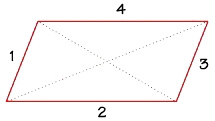For the sizes of the interior angles of the quadrilateral ABCD, the following applies: the angle alpha is 26° greater than the angle beta, twice the angle Beta is 5° less than the angle gamma, and the angle gamma is 36° greater than the angle delta. Determine the angles.

A =  86
B =  60
C =  125
D =  89

### Step-by-step explanation:

A = 26 + B
2·B = C-5
C = 36 + D
A+B+C+D = 360

A-B = 26
2B-C = -5
C-D = 36
A+B+C+D = 360

Row 4 - Row 1 → Row 4
A-B = 26
2B-C = -5
C-D = 36
2B+C+D = 334

Row 4 - Row 2 → Row 4
A-B = 26
2B-C = -5
C-D = 36
2C+D = 339

Pivot: Row 3 ↔ Row 4
A-B = 26
2B-C = -5
2C+D = 339
C-D = 36

Row 4 - 1/2 · Row 3 → Row 4
A-B = 26
2B-C = -5
2C+D = 339
-1.5D = -133.5

D = -133.5/-1.5 = 89
C = 339-D/2 = 339-89/2 = 125
B = -5+C/2 = -5+125/2 = 60
A = 26+B = 26+60 = 86

A = 86
B = 60
C = 125
D = 89

Our linear equations calculator calculates it.Did you find an error or inaccuracy? Feel free to write us. Thank you!

Tips for related online calculators
Do you have a system of equations and looking for calculator system of linear equations?